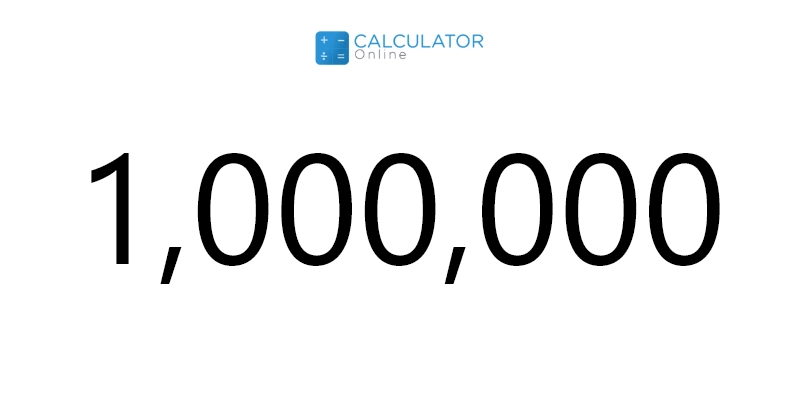• Hire UsUh Oh! It seems you’re using an Ad blocker!

We always struggled to serve you with the best online calculations, thus, there's a humble request to either disable the AD blocker or go with premium plans to use the AD-Free version for calculators.

Or# 1 Million in Numbers

## What is 1 million in numbers?

“If we express a number by thousand-thousandth of one then it becomes one million in numbers”

In the early times, the word “million” was derived from Italian (milione in modern Italian) which means mille, “thousand”, and the augmentative suffix -one. A million in numbers is abbreviated as “m”. One million (1,000,000) is the natural number (or counting number) followed by 999,999 and preceded by 1,000,001.

Example:

How much is a million of 30?

30 million is equal to 30000000.

### How to write one million in numbers?

If you want to know how do you write 1 million. Follow the steps to write 1 million number form that are given below as:
Step #1:
• To write one million numbers, firstly delete the name “million” and then multiply 1 by 10^6.
Step #2:
• After that, move the decimal separately 6 places to the left sides:

1 × 10^6 = 1000000,

1 → 10 → 100 → 1,000 → 10,000 → 100,000 → 1,000,000.

From the above value, you can also estimate how many zeros in 1 million are.
Also, you can calculate how much is 1 million in numbers and convert it into different currency formats by using an online  swiftly.

In the numeral system, the terms rupees, lakhs, and millions are used to understand and  write a large amount easier. The place value of digits are managed in International numeral systems in order of Ones, Tens, Hundreds, Thousands, Ten Thousand, Hundred Thousand, Millions, Billions, and so on.

### 1 Million in Rupees:

To convert the 1 in a million in rupees, firstly take one million as ten lakhs in rupees. As you know one lakhs has six zeros digits. Let’s see the value of millions or 1 million in rupees given in the below table:
 Millions Rupees 1 1000000 2 2000000 10 10000000 50 50000000 60 60000000 70 70000000 100 100000000 1000 1000000000

### 1 Million How Many Lakhs:

The representation of amounts from millions to lakhs change from the International to the numeral system can be written and is given below as:

i.e. 1 Million = 10 Lakhs

 Millions Lakhs 1 10 (= 1000000) 2 20 (= 2000000) 5 50 (= 5000000) 10 100 (= 10000000) 20 200 (= 20000000) 50 500 (= 50000000)

### 1 Million How Many Crores:

The currency change from millions to crores in which the conversion takes from the International to the numeral system. The expression of 1 million in numbers to crore is given as:

 Millions Crores 1 0.1 10 1 20 2 30 3

### 1 million dollars in numbers:

One million dollars number converts from million to the dollar in the International numeral system. Let's see the given below table and identify 1million dollar in rupees. These include:
 Millions Rupees 1 1000000 2 2000000 3 3000000

### How much is a million?

Now you must know how much is 1 million in the currency system. The answer to this question can be described in different ways. As you know the amount of money is always read in units, ten, hundred, thousand and so on. Generally, the word “Million” is used to describe large amounts of money in the international currency system. However, the value of 1 million in numbers can be expressed as:

1 Million = 1000000 rupees

1 Million = 10 lakhs

1 Million = 0.1 crore

### Solved examples:

Example 1:

Express 21 million in lakhs.

Solution:

We know that 1 Million = 10 lakhs

21 million = 21 × 10 lakhs

21 millions = 210 lakhs

Example 2:

Write the value of 35 million in crores.

Solution:

We know that 1 Million = 0.1 crore

35 millions = 35 × 0.1 crore

35 millions = 3.5 crores

Example 3:

Convert 1million dollar in rupees.

Solution:

As we know one million number = 1,000,000 rupees

1 million in dollar = 4810.12 (US)

1,000,000 * 4810.12

one million dollars number = 4,810,120,000 rupees.